### Practice Set 3.2 Circle Class 10th Mathematics Part 2 MHB Solution

Practice Set 3.2

1. Two circles having radii 3.5 cm and 4.8 cm touch each other internally. Find the…
2. Two circles of radii 5.5 cm and 4.2 cm touch each other externally. Find the distance…
3. If radii of two circles are 4 cm and 2.8 cm. Draw figure of these circles touching each…
4. In fig 3.27, the circles with centres P and Q touch each other at R. A line passing…
5. In fig 3.28 the circles with centres A and B touch each other at E. Line is a common…

###### Practice Set 3.2
Question 1.

Two circles having radii 3.5 cm and 4.8 cm touch each other internally. Find the distance between their centres.

Given: Two circles are touching each other internally.

∵The distance between the centres of the circles touching internally is equal to the difference of their radii.

⇒distance between their centres = 4.8 cm – 3.5 cm = 1.3 cm

Question 2.

Two circles of radii 5.5 cm and 4.2 cm touch each other externally. Find the distance between their centres.

Given: Two circles are touching each other externally

We know that if the circles touch each other externally, distance between their centres is equal to the sum of their radii.

⇒distance between their centres = 5.5 cm + 4.2 cm = 9.7 cm

Question 3.

If radii of two circles are 4 cm and 2.8 cm. Draw figure of these circles touching each other –

(i) externally

(ii) internally.

(i)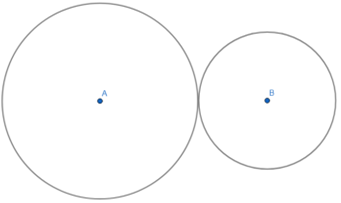Steps of construction:

1. Draw a circle with radius 4cm and centre A.

2. Draw another circle with radius 2.8 cm and centre B such that they touch each other externally.

(ii)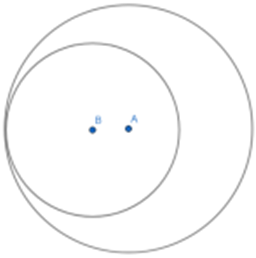Steps of construction:

1. Draw a circle with radius 4cm and centre A.

2. Draw another circle with radius 2.8 cm and centre B such that they touch each other internally.

Question 4.

In fig 3.27, the circles with centres P and Q touch each other at R. A line passing through R meets the circles at A and B respectively. Prove that -

(1) seg AP || seg BQ,

(2) ∆ APR ~ ∆ RQB, and

(3) Find ∠ RQB if ∠ PAR = 35°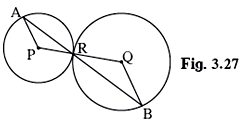(1)In ΔAPR,

AP = RP {Radius of the circle with centre P}

∠PAR = ∠PRA … (1)

In ΔRQB,

RQ = QB {Radius of the circle with centre Q}

∠QRB = ∠QBR …. (2)

⇒ ∠PRA = ∠QRB {Vertically Opposite Angle} ….(3)

⇒ ∠PAR = ∠QBR {From (1), (2) and (3)}

⇒ Alternate interior angles are equal.

⇒ AP || BQ

Hence, proved.

(2) In ∆ APR and ∆ RQB,

∠PAR = ∠QBR and ∠PRA = ∠QRB {From (1) and (2)}

⇒ ∆ APR ~ ∆ RQB {AA}

Hence, proved.

(4) Given: ∠ PAR = 35°

⇒ ∠QBR = 35° = ∠QRB {Proved previously}

In ∆ RQB,

⇒ ∠ RQB + ∠ QRB + ∠QBR = 180° {Angle sum property of the triangle}

⇒∠ RQB + 35° + 35° = 180°

⇒∠ RQB = 180°- 70° = 110°

Question 5.

In fig 3.28 the circles with centres A and B touch each other at E. Line is a common tangent which touches the circles at C and D respectively. Find the length of seg CD if the radii Fig. 3.28 of the circles are 4 cm, 6 cm.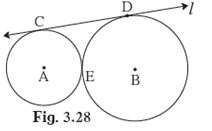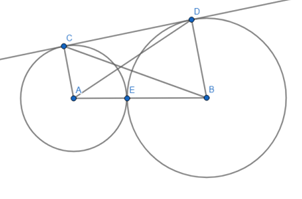Given that two circles with centre A and B touch each other externally. We know that if the circles touch each other externally, distance between their centres is equal to the sum of their radii.

⇒AB = (4 + 6) cm = 10 cm

In ∆ABC right-angles at A,

BC2 = CA2 + AB2 {Using Pythagoras theorem}

⇒BC2 = 42 + 102

⇒BC2 = 16 + 100

⇒ BC = √116 cm

In ∆DBC,

∠ BDC = 90° because D is the point of contact of tangent CD to circle centred B

BC2 = CD2 + DB2 {Using Pythagoras theorem}

⇒CD2 = 116 - 62

⇒CD2 = 116 - 36

⇒ CD = √80 cm = 4√5

## PDF FILE TO YOUR EMAIL IMMEDIATELY PURCHASE NOTES & PAPER SOLUTION. @ Rs. 50/- each (GST extra)

SUBJECTS

HINDI ENTIRE PAPER SOLUTION

MARATHI PAPER SOLUTION
SSC MATHS I PAPER SOLUTION
SSC MATHS II PAPER SOLUTION
SSC SCIENCE I PAPER SOLUTION
SSC SCIENCE II PAPER SOLUTION
SSC ENGLISH PAPER SOLUTION
SSC & HSC ENGLISH WRITING SKILL
HSC ACCOUNTS NOTES
HSC OCM NOTES
HSC ECONOMICS NOTES
HSC SECRETARIAL PRACTICE NOTES

2019 Board Paper Solution

HSC ENGLISH SET A 2019 21st February, 2019

HSC ENGLISH SET B 2019 21st February, 2019

HSC ENGLISH SET C 2019 21st February, 2019

HSC ENGLISH SET D 2019 21st February, 2019

SECRETARIAL PRACTICE (S.P) 2019 25th February, 2019

HSC XII PHYSICS 2019 25th February, 2019

CHEMISTRY XII HSC SOLUTION 27th, February, 2019

OCM PAPER SOLUTION 2019 27th, February, 2019

HSC MATHS PAPER SOLUTION COMMERCE, 2nd March, 2019

HSC MATHS PAPER SOLUTION SCIENCE 2nd, March, 2019

SSC ENGLISH STD 10 5TH MARCH, 2019.

HSC XII ACCOUNTS 2019 6th March, 2019

HSC XII BIOLOGY 2019 6TH March, 2019

HSC XII ECONOMICS 9Th March 2019

SSC Maths I March 2019 Solution 10th Standard11th, March, 2019

SSC MATHS II MARCH 2019 SOLUTION 10TH STD.13th March, 2019

SSC SCIENCE I MARCH 2019 SOLUTION 10TH STD. 15th March, 2019.

SSC SCIENCE II MARCH 2019 SOLUTION 10TH STD. 18th March, 2019.

SSC SOCIAL SCIENCE I MARCH 2019 SOLUTION20th March, 2019

SSC SOCIAL SCIENCE II MARCH 2019 SOLUTION, 22nd March, 2019

XII CBSE - BOARD - MARCH - 2019 ENGLISH - QP + SOLUTIONS, 2nd March, 2019

HSC Maharashtra Board Papers 2020

(Std 12th English Medium)

HSC ECONOMICS MARCH 2020

HSC OCM MARCH 2020

HSC ACCOUNTS MARCH 2020

HSC S.P. MARCH 2020

HSC ENGLISH MARCH 2020

HSC HINDI MARCH 2020

HSC MARATHI MARCH 2020

HSC MATHS MARCH 2020

SSC Maharashtra Board Papers 2020

(Std 10th English Medium)

English MARCH 2020

HindI MARCH 2020

Hindi (Composite) MARCH 2020

Marathi MARCH 2020

Mathematics (Paper 1) MARCH 2020

Mathematics (Paper 2) MARCH 2020

Sanskrit MARCH 2020

Important-formula

THANKS